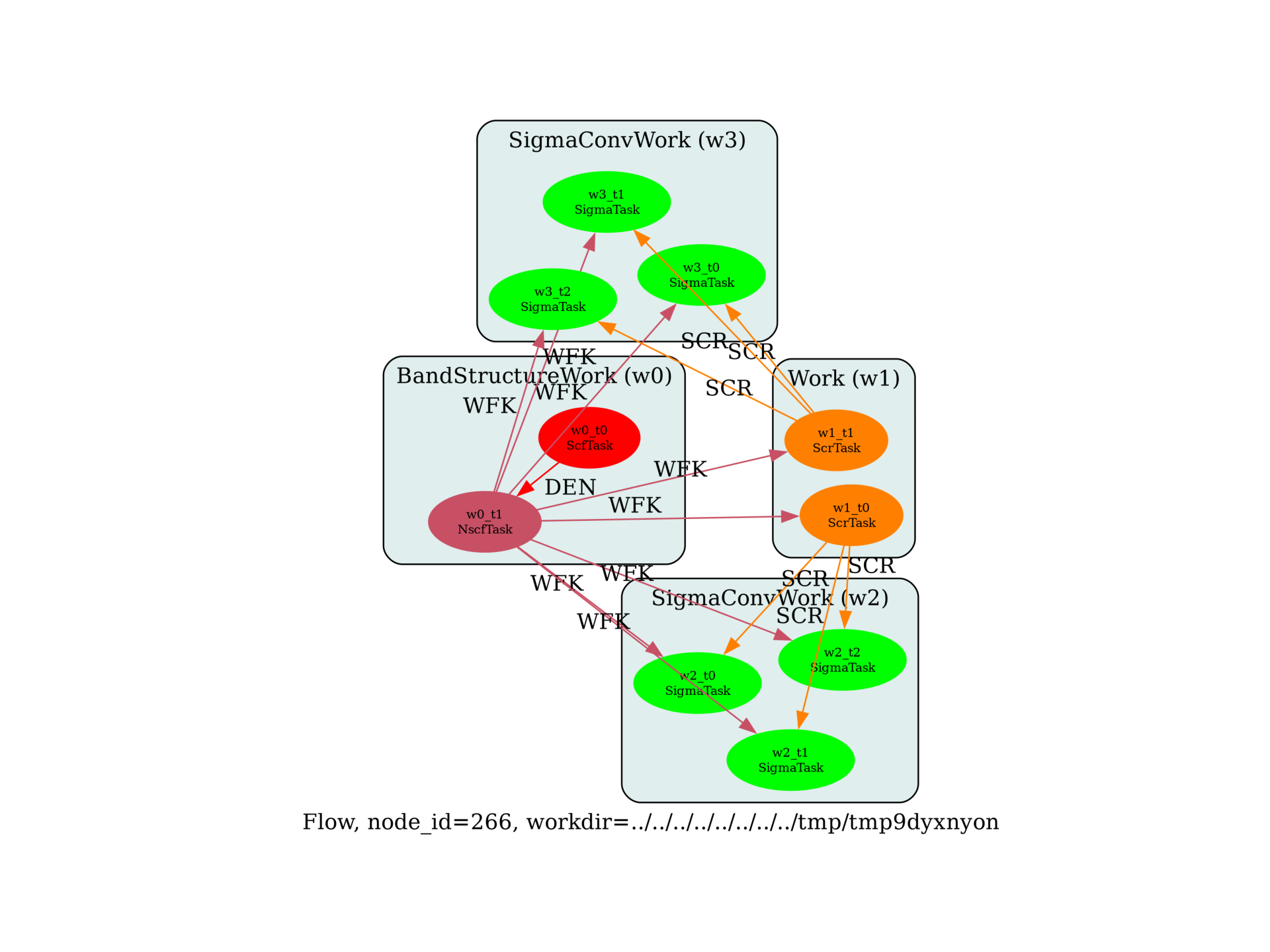G0W0 convergence study¶

G0W0 convergence study wrt ecuteps and the number of bands in W.import sys
import os
import numpy as np

import abipy.abilab as abilab
import abipy.data as abidata
import abipy.flowtk as flowtk

def make_inputs(paral_kgb=1):
"""
Returns a tuple of 4 input files for SCF, NSCF, SCR, SIGMA calculations.
These files are then used as templates for the convergence study
wrt ecuteps and the number of bands in W.
"""
multi = abilab.MultiDataset(abidata.structure_from_ucell("SiC"),
pseudos=abidata.pseudos("14si.pspnc", "6c.pspnc"), ndtset=4)

ecut = 12
global_vars = dict(
ecut=ecut,
istwfk="*1",
paral_kgb=paral_kgb,
gwpara=2,
iomode=1,
)

ecuteps = 4
ngkpt = [4, 4, 4]
shiftk = [0, 0, 0]

multi.set_vars(global_vars)
multi.set_kmesh(ngkpt=ngkpt, shiftk=shiftk)

# SCF
multi.set_vars(
nband=10,
tolvrs=1.e-8,
)

# NSCF
multi.set_vars(
nband=25,
tolwfr=1.e-8,
iscf=-2
)

# SCR
multi.set_vars(
optdriver=3,
ecutwfn=ecut,
nband=20,
symchi=1,
inclvkb=0,
ecuteps=ecuteps,
)

# SIGMA
multi.set_vars(
optdriver=4,
nband=20,
ecutwfn=ecut,
ecutsigx=ecut,
#ecutsigx=(4*ecut), ! This is problematic
symsigma=1,
ecuteps=ecuteps,
)

multi.set_kptgw(kptgw=[[0,0,0], [0.5, 0, 0]], bdgw=[1, 8])

return multi.split_datasets()

def build_flow(options):
# Working directory (default is the name of the script with '.py' removed and "run_" replaced by "flow_")
if not options.workdir:
options.workdir = os.path.basename(sys.argv).replace(".py", "").replace("run_", "flow_")

# Get our templates
scf_inp, nscf_inp, scr_inp, sig_inp = make_inputs()

ecuteps_list = np.arange(2, 8, 2)
max_ecuteps = max(ecuteps_list)

flow = flowtk.Flow(workdir=options.workdir, manager=options.manager)

# Band structure work to produce the WFK file
bands = flowtk.BandStructureWork(scf_inp, nscf_inp)
flow.register_work(bands)

# Build a work made of two SCR runs with different value of nband
# Use max_ecuteps for the dielectric matrix (sigma tasks will
# read a submatrix when we test the convergence wrt to ecuteps.
scr_work = flowtk.Work()

for inp in scr_inp.generate(nband=[10, 15]):
inp.set_vars(ecuteps=max_ecuteps)

flow.register_work(scr_work)

# Do a convergence study wrt ecuteps, each work is connected to a
# different SCR file computed with a different value of nband.

# Build a list of sigma inputs with different ecuteps
sigma_inputs = list(sig_inp.generate(ecuteps=ecuteps_list))

sigma_inputs=sigma_inputs)
flow.register_work(sigma_conv)

return flow

# This block generates the thumbnails in the AbiPy gallery.
# You can safely REMOVE this part if you are using this script for production runs.
__name__ = None
import tempfile
options = flowtk.build_flow_main_parser().parse_args(["-w", tempfile.mkdtemp()])
build_flow(options).graphviz_imshow()

@flowtk.flow_main
def main(options):
"""
This is our main function that will be invoked by the script.
flow_main is a decorator implementing the command line interface.
Command line args are stored in `options`.
"""
return build_flow(options)

if __name__ == "__main__":
sys.exit(main())

Total running time of the script: ( 0 minutes 0.746 seconds)

Gallery generated by Sphinx-Gallery